# Reversing the Stryd — linear models

Predicting running powerMisha Iakovlev and Matt Squire

Oct 30, 2020 8 min read

The code and data used for the analysis can be found on Github.

This blog is part of our ongoing series documenting Wearable My Foot, our ongoing R&D project to build a wearable fitness device powered by data science and machine learning. Today we’re looking at exercise data from Stryd, a commercially-available fitness tracker for runners.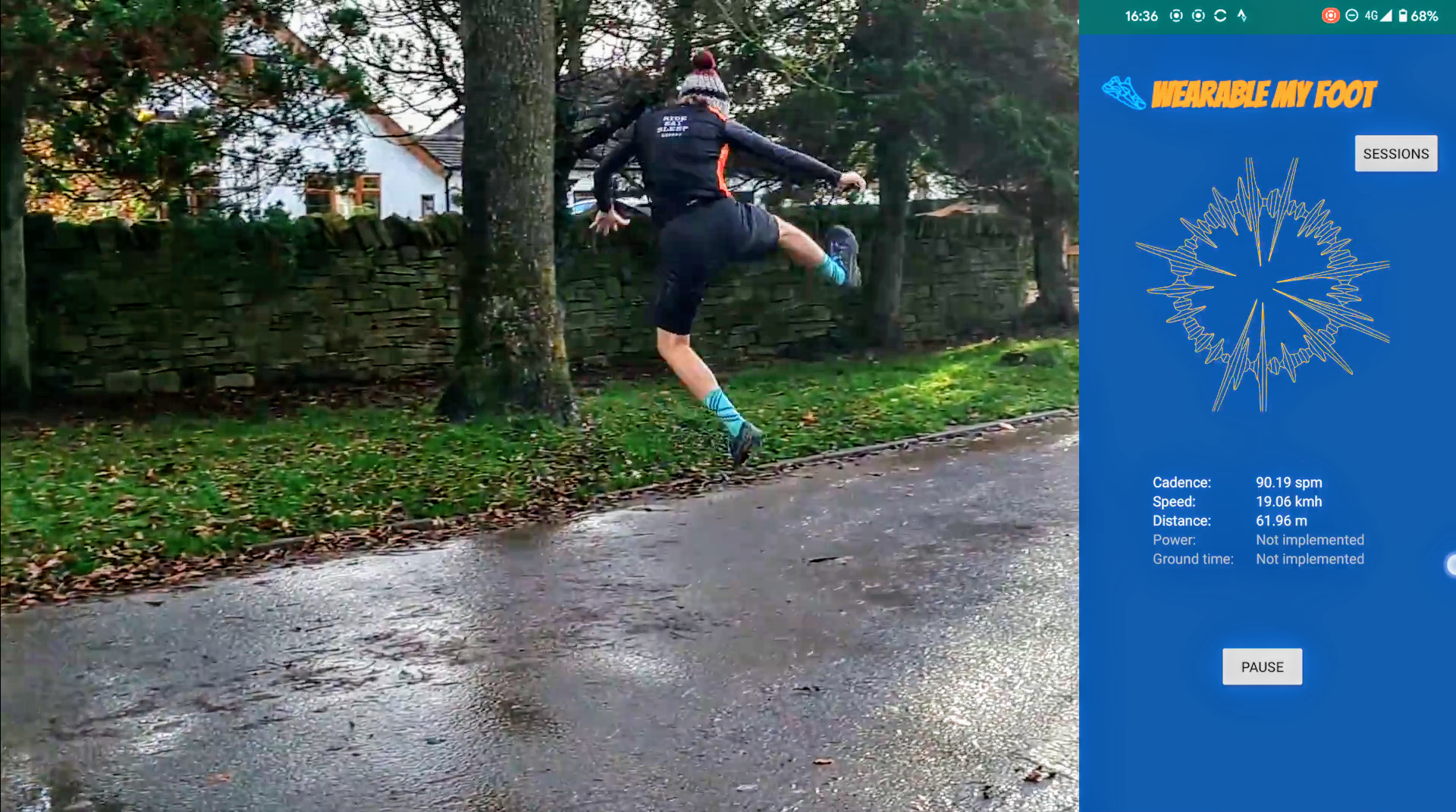Stryd accurately records speed, cadence and running power over the course of a run. Because running power is a particularly useful metric for serious runners, we want to extract raw data from Stryd and understand how running power is related to other metrics, such as pace and cadence.

In a previous blog post we started to build a procedure from the ground up using a physical model called EESA. Although the model seems complex, it boils down to a few key inputs, like horizontal and vertical speed, and some reasonable assumptions about the physics behind running. This model is great in theory but presents some difficulties when faced with the realities of noisy sensor data. This time, we take a step back from the physics and see if we can ‘blindly’ model running power by analysing correlations between the values Stryd outputs.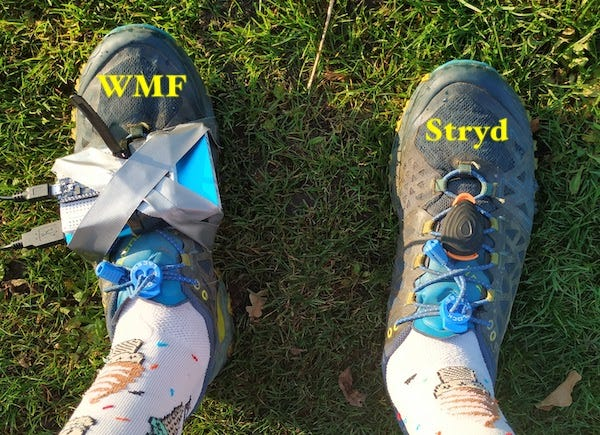## Data and metrics

To begin with, let’s go through what Stryd gives us to work with. Units for each variable are given in brackets.

• Power (W) — running power estimate; this is what we want to estimate.
• Cadence (spm) — number of steps/strides per minute.
• Vertical oscillation — amplitude of oscillation of a foot along the vertical axis, or in other words how high the foot rises at its peak.
• Stance time (ms) — the duration of time the foot is on the ground (sometimes called ground time or contact time).
• Air power (W) — estimated power of air resistance.
• Leg spring stiffness (N/m) — Stryd models the leg as a compressed spring. The ‘spring stiffness’ measures how well a runner recycles energy as their foot hits the ground (https://blog.stryd.com/2019/12/05/the-secret-of-running-run-smart-to-run-faster).
• Speed (m/s)
• Altitude (m)
• Distance (m)

Ultimately all of these metrics are derived from a small number of sensors, including an accelerometer, gyroscope, altimeter, and wind meter. Except for the last two, we are using all the metrics as is. Altitude and distance, on the other hand, are absolute quantities that depend on time, and in those cases we’re really interested in is the differential— the change in the variable over time.

## Data collection

For our experiments, we have recorded several runs under different conditions:

• Straight line and non-straight line paths
• Running on flat and inclined surfaces

Giving us 1138 data points (measurements) in total. However, we remove all data points where measured power is close to zero, under the assumption that the runner was standing still at these times, which brings us down to 949 data points to experiment with.

## Exploratory analysis

First of all we want to see if the data that we will use to fit the model makes sense, i.e. does it show correlation where we would expect them, as well as ensuring that there’s not too much noise in the data.

To start with let’s plot all pairings of these variables to see if any patterns jump out.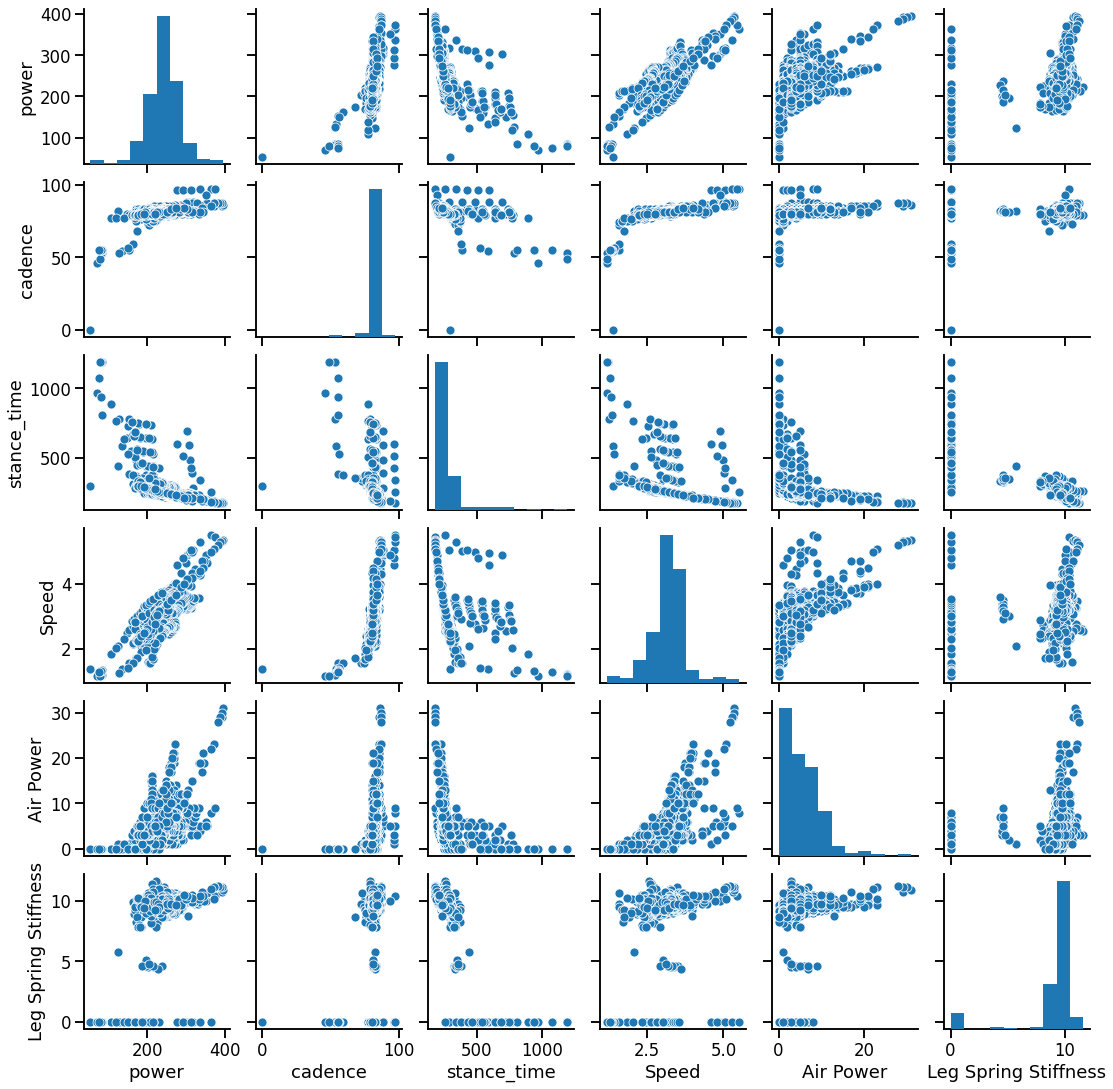A pair plot of a selection of variables in the data

We’re looking for any variables that correlate strongly with power. For instance, the speed-power graph looks like we can draw a straight line through it and that suggests that speed is a good predictor of power.

We get a clearer picture by plotting the matrix of correlation coefficients, which indicate how strongly two variables are correlated. For instance, if the coefficient for speed with power is 0.8, then we know that speed is 80% correlated with power.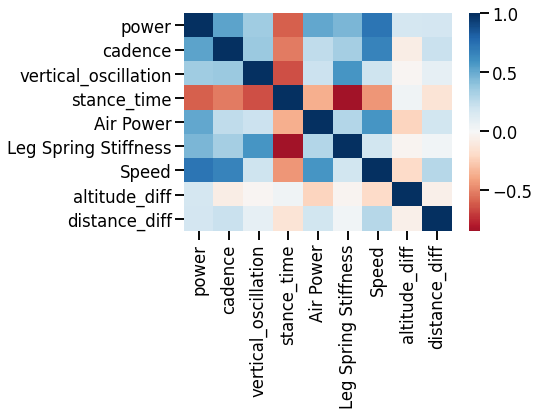Correlation matrix

Some variables have strong correlation to power: namely cadence, stance time, air power and speed; all of which are expected according to EESA model.

Now that we have a broad idea of what kinds of correlation to expect, now we can try fitting a number of different models. From here on it gets quite detailed, so feel free to skip to the conclusions at the end.

## Experimental design

For this experiment we are trying to fit a linear model i.e. an equation of the form `y = sum(w_i * x_i) + b` , where y is our target variable (power), `x_i` and `w_i` are the features and the weight associated with them, and `b` is the intercept. (weights are sometimes called coefficients when talking about linear models).

Put visually, rather than algebraically, we want to find a straight line that fits the data as closely as possible, under the assumption that it is indeed possible to fit a line to the data at all. We’ll need to try a few different approaches and test their accuracy.

### Feature engineering

The target output is power, but we need to decide what input features to use. It makes sense to narrow the original features down to only those that we expect to be used in power calculation from the EESA model, and that we are potentially able to calculate from acceleration:

• Vertical oscillation
• Stance time
• Speed

An extra quirk of the EESA model is that we expect power to be related to the square of speed, rather than the speed itself. Therefore we’ll create some derived features that allow us to include things like speed squared.

Feature engineering is a big topic in data science. There are many articles on this topic, for instance https://towardsdatascience.com/feature-engineering-for-machine-learning-3a5e293a5114.

### Performance assessment

To assess the performance of our models, we are using two metrics. First, the coefficient of determination, or R², is a proportion of the target variance that can be predicted from the input features (values closer to 1.0 are better), which is a standard metric to assess linear regression performance. Second, mean absolute error, MAE, which serves as an intuitive measure of how much we deviate from the expected value for running power.

As is common practice we assess the performance by splitting the data into a training and a testing sets, fitting a model on the training data, and calculating metrics using the testing and predicted values of power. However, a single train-test split might not be representative of the underlying data (due to a random nature of splitting). We can use 5-fold cross-validation instead: split data into 5 chunks of the same size, fit 5 models using each chunk for testing once, and taking mean average of the performance metrics.

## Linear regression and Ordinary Least Squares

The simplest variant of linear regression fitting method is called Ordinary Least Squares. This method minimises the residual sum of squares between the observed target (i.e. the measurements of power according to Stryd) and the values predicted by a model.

Fitting linear regression with all three feature sets, we get the following results:

mae_mean r2_mean
Original -19.112 0.60626
Selected -23.658 0.384907
Polynomial -21.7786 0.25584

The results show the best performance for a model fitted with the original features (according to both metrics), suggesting that our feature engineering attempts might not be beneficial for predicting our target.

## Regularisation

Beyond Ordinary Least Squares there are a set of methods that use *regularisation. *Regularisation aims to avoid a model overfitting, which it does by constraining the learned coefficients.

### Ridge

Ridge can be thought of as an extension of Ordinary Least Squares, that, in addition to minimising the error, also applies a penalty for the size of the coefficients (w_i). In contrast with OLS, Ridge requires the complexity parameter alpha (how much the size of coefficients should be penalised). We do not have a prior knowledge of what is the best value for alpha in our case, so we have to perform the parameter tuning. Thankfully, we only have one parameter to tune, so we can simply take a range of alpha values and pick one that gives the best performance.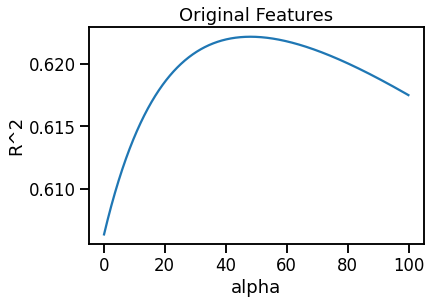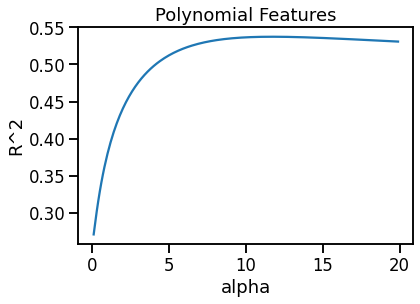We fit Ridge linear regression using the original and polynomial features.

In both cases we see an improvement in performance, in respect to the performance of OLS. The improvement is more significant for the set of polynomial features bringing it from R² value 0.26 to 0.54.

mae_mean r2_mean
Original -19.1893 0.618525
Polynomial -20.2247 0.537264

### Lasso

Another regularisation option that we have is Lasso, that penalises for non-zero coefficients, effectively trying to find a model that can explain a target with the smallest number of features.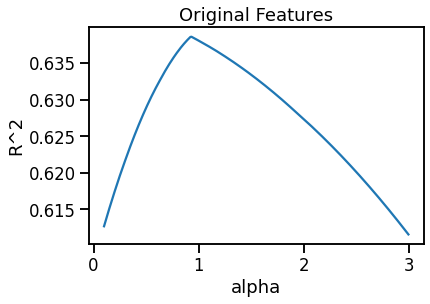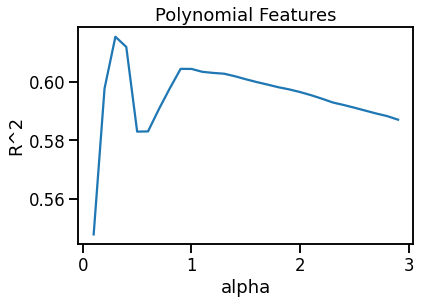As with the previous methods, we fit it with the original and the polynomial features. In both cases, it gives even greater improvement in performance than Ridge, resulting in the Lasso model with the original feature set the overall best performance model.

mae_mean r2_mean
Original -19.0823 0.638604
Polynomial -19.2318 0.615413

## Results

As mentioned previously the overall top performing model is given by Lasso fitted with the original features (R² = 0.64, MAE = 19.08). Let’s explore this model further.

mae_mean r2_mean
Ordinary Least Squares -19.112 0.60626
Ordinary Least Squares (Selected) -23.658 0.384907
Ordinary Least Squares (Polynomial) -21.7786 0.25584
Ridge -19.1893 0.618525
Ridge (Polynomial) -20.2247 0.537264
Lasso -19.0823 0.638604
Lasso (Polynomial) -19.2318 0.615413

The table below shows non-zero coefficients.

Feature Coefficient
Vertical Oscillation 1.2142
Stance Time -2.23361
Air Power 4.12725
Leg Spring Stiffness 10.407
Speed 28.0068
Altitude Diff 13.6
Distance Diff -0.652722

The fitted coefficients align with our expectations:

• Power is greater for greater vertical oscillation amplitude, speed, air power, and incline gradient
• Low stance time and high leg spring stiffness (which are negatively correlated to each other) mean greater power

However, only a subset of these features can be (potentially) inferred purely from acceleration: vertical oscillation, stance time, speed and change in distance. Constructing a model with only this variables (their respective coefficients) gives us a good starting point for calculating power using raw accelerometer data.

This approach, however, has a few limitations:

• Data collection: the range of different conditions that we captured is somewhat limited, included the limited range of power values, and this linear model, most probably, will not account for the cases under severely different conditions.
• The Stryd’s power measurement depends on the speed, both according to correlation and the fitted model, rather than on the square of speed, which we expect from the EESA. Which might indicate some bias, that we, so far, were not able to capture or discover.
• We will not be able to infer from acceleration some of the features (such as air power) that have a high influence on total power.

## Conclusions

With very little attention being paid to the underlying physics we’re able to estimate power somewhat accurately through a linear model. However we would still need to calculate certain values that are not easily derived from acceleration alone.

Speaking more broadly, the Wearable My Foot project can currently work out step counts and speed/cadence using acceleration in combination with some clever numerical techniques. While the approach described in this article allows us to estimate running power over short time windows, it turns out to break down when faced with longer time periods that involve a lot of elevation change. Further research is needed before we can give a proper account of running power.